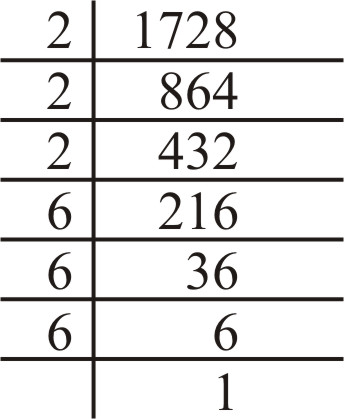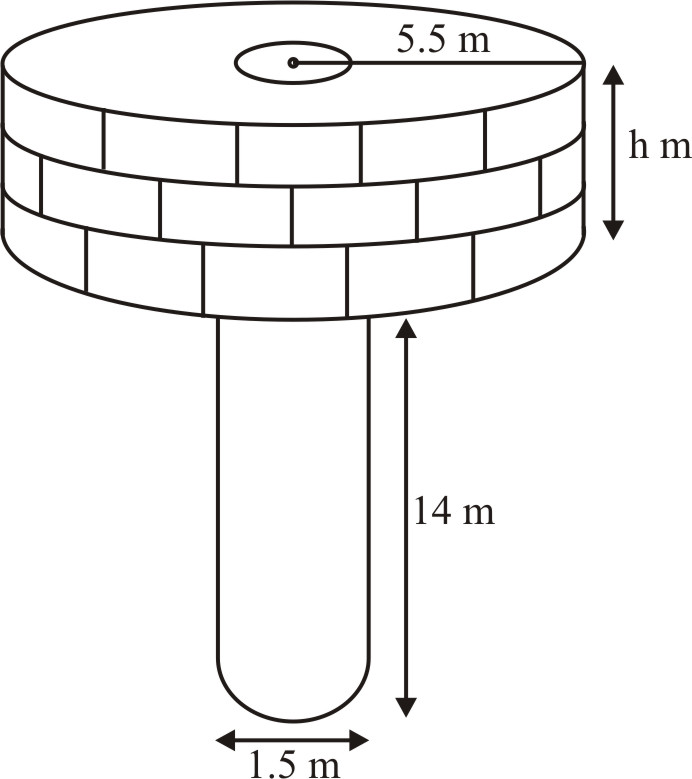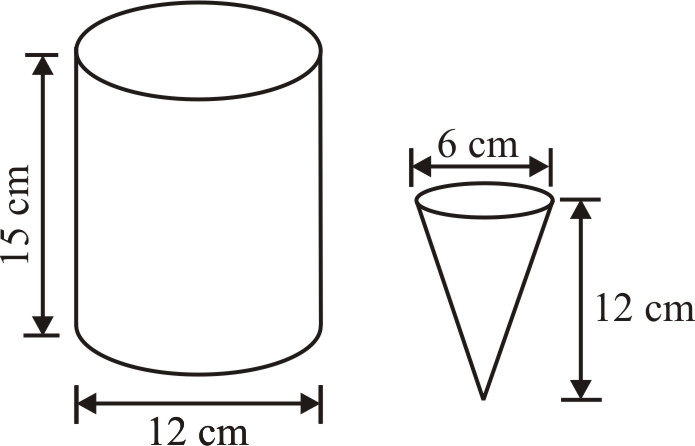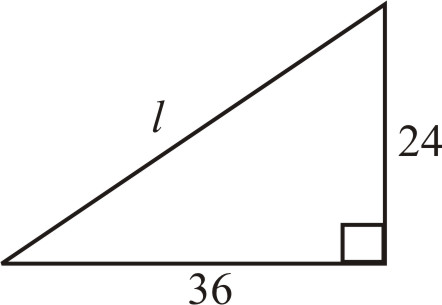# Surface Area and Volume : Exercise - 13.3 (Mathematics NCERT Class 10th)

Take $\pi = {{22} \over 7}$, unless stated otherwise.

Q.1      A metallic sphere  of radius 4.2 cm is melted and recast into the shape of a cylinder of radius 6 cm. Find the height  of the cylinder.
Sol.

Volume of the sphere
$= {4 \over 3}\pi {r^3} = {4 \over 3} \times \pi \times {\left( {4.2} \right)^3}c{m^3}$
If h is the  height of a cylinder of radius  6 cm. Then its volume,
$= \pi {\left( 6 \right)^2}h\,c{m^3} = 36\,\pi h\,c{m^3}$
Since, the volume of metal in the form of sphere  and cylinder remains the same , we have
$36\,\pi h = {4 \over 3} \times \pi \times 4.2 \times 4.2 \times 4.2$
$\Rightarrow$    $h = {1 \over {36}} \times {4 \over 3} \times 4.2 \times 4.2 \times 4.2$
$\Rightarrow$    h = 2.744

Q.2      Metallic  spheres of radii 6 cm, 8 cm and 10 cm, respectively, are melted to form a single solid sphere. Find  the radius of the resulting sphere.
Sol.

Sum of the volumes of 3 gives spheres.
$= {4 \over 3}\pi \left( {{r_1}^3 + {r_2}^3 + {r_3}^3} \right)$
$= {4 \over 3}\pi \left( {{6^3} + {8^3} + {{10}^3}} \right)c{m^3}$
$= {4 \over 3}\pi \left( {216 + 512 + 1000} \right)c{m^3}$
$= {4 \over 3}\pi \left( {1728} \right)c{m^3}$
Let R be  the radius of the new spheres whose volume is the sum of the  volumes of 3 given spheres.
Therefore      $= {4 \over 3}\pi {R^3} = {4 \over 3}\pi \left( {1728} \right)$
$\Rightarrow$    ${R^3} = 1728$
$\Rightarrow$    ${R^3} = {\left( {12} \right)^3}$
$\Rightarrow$    R = 12
Hence, the  radius of the  resulting sphere is 12 cm.Therefore 1728 = 23 × 63 = (2 × 6)3 = 12)3

Q.3      A 20 cm deep well with  diameter 7 cm is dug and the  earth  from  digging is evenly spread out to form a platform 22 m by 14 m. Find the  height of the platform.
Sol.

Let h m be the  required height  of the platform.
The shape of the platform will be like the shape of a cuboid 22 m × 14 m × h with a hole  in the  shape of cylinder of radius  3.5 m and depth h m.
The volume  of the platform will be equal to the  volume  of the  earth  dug out from  the well.
Now, the  volume  of the earth = Volume  of the cylindrical well
$= \pi {r^2}h$
$= {{22} \over 7} \times 12.25 \times 20\,{m^3}$
$= 770\,{m^3}$
Also, the  volume of the platform = 22 × 14 × h ${m^3}$
But volume of the platform = Volume of the  well
i.e.,      22 × 14 × h = 770
$h = {{770} \over {22 \times 14}} = 2.5$
Therefore  Height of the platform = 2.5 m

Q.4     A well of diameter 3 m is dug 14 m deep. The earth taken out of it has  been spread evenly all around it in the shape of a circular  ring  of width 4 m to form an embankment. Find the height of the embankment.
Sol.

Let h be the required height of the embankment.
The shape of the embankment will be like the shape of a cylinder of internal radius 1.5 m and  external radius (4 + 1.5)m = 5.5 m (see figure).The  volume of the embankment will be equal  to the volume of earth  dug out from the  well.
Now,  the volume of the earth = Volume  of the cylindrical well
$= \pi \times {\left( {1.5} \right)^2} \times 14\,{m^3} = 31.5\,\pi \,{m^3}$
Also  the volume of the embankment
$= \pi \left( {{{5.5}^2} - {{1.5}^2}} \right)h\,{m^3}$
$= \pi \left( {5.5 + 1.5} \right)\left( {5.5 - 1.5} \right)h\,{m^3}$
$= \pi \times 7 \times 4h\,{m^3} = 28\,\pi h\,{m^3}$
Hence, we have
$28\,\pi h = 31.5\,\pi$
$\Rightarrow$    $h = {{31.5} \over {28}} = 1.125$
Hence, the required height  of the  embankment = 1.125 m

Q.5     A container shaped like a right  circular  cylinder having diameter 12 cm and height 15 cm is full of ice cream. The ice cream is to be  filled into cones of height12 cm and diameter 6 cm, having  hemispherical shape on the top. Find the number of such  cones which  can be  filled with ice cream.
Sol.

Volume of the cylinder
$= \pi {r^2}h$
$= \pi {\left( {{{12} \over 2}} \right)^2} \times 15$
$= \pi \times {6^2} \times 15$Volume  of a cone having  hemispherical shape on the top
$= {1 \over 3}\pi {r^2}h + {2 \over 3}\pi {r^3} = {1 \over 3}\pi {r^2}\left( {h + 2r} \right)$
$= {1 \over 3}\pi {\left( {{6 \over 2}} \right)^2}\left( {12 + 2 \times {6 \over 2}} \right)$
$= {1 \over 3}\pi \times {3^2} \times 18$
Let the number of cone that can be filled with ice cream be h.
Then ${1 \over 3}\pi \times {3^2} \times 18 \times n = \pi \times {6^2} \times 15$
$n = {{\pi \times 6 \times 6 \times 15} \over {\pi \times 3 \times 3 \times 18}} \times 3 = 10$
Q.6 How many silver  coins, 1.75 cm in diameter and of  thickness 2 mm, must be melted to form a cuboid of dimensions 5.5 cm × 10 cm × 3.5 cm?
Sol. The shape of the coin will be like the shape of a cylinder of radius ${{1.75} \over 2}cm$
= 0.875 cm and of height 2mm $= {2 \over {10}}cm = .2\,cm$
Its volume
$= \pi {r^2}h = {{22} \over 7} \times 0.875 \times 0.875 \times .2\,c{m^3}$
$= 0.48125\,c{m^3}$
Volume  of the cuboid $= 5.5 \times 10 \times 3.5\,c{m^3} = 192.5\,c{m^3}$
Number  of coins  required to form the cuboid
$= {{Volume\,of\,the\,cuboid} \over {Volume\,of\,the\,coin}} = {{192.5} \over {0.48125}} = 400$
Hence, 400  coins must be melted to form a cuboid.

Q.6     How many silver  coins, 1.75 cm in diameter and of  thickness 2 mm, must be melted to form a cuboid of dimensions 5.5 cm × 10 cm × 3.5 cm?
Sol.

The shape of the coin will be like the shape of a cylinder of radius ${{1.75} \over 2}cm$
= 0.875 cm and of height 2mm $= {2 \over {10}}cm = .2\,cm$
Its volume
$= \pi {r^2}h = {{22} \over 7} \times 0.875 \times 0.875 \times .2\,c{m^3}$
$= 0.48125\,c{m^3}$
Volume  of the cuboid $= 5.5 \times 10 \times 3.5\,c{m^3} = 192.5\,c{m^3}$
Number  of coins  required to form the cuboid
$= {{Volume\,of\,the\,cuboid} \over {Volume\,of\,the\,coin}} = {{192.5} \over {0.48125}} = 400$
Hence, 400  coins must be melted to form a cuboid.

Q.7     A cylindrical  bucket, 32 m high  and with  radius of base 18 cm, is filled with sand. The bucket  is emptied on the ground and a conical heap of sand is formed. If the height of the conical heap is 24 cm, find the radius  and slant height  of the heap.
Sol.

Volume of the  sand = Volume of the cylindrical bucket
$= \pi {r^2}h = \pi \times 18 \times 18 \times 32\,c{m^3}$Volume of the conical heap
$= {1 \over 3}\pi {r^2}h$, where  r = ? , h = 24 cm
$= {1 \over 3}\pi {r^2} \times 24\,c{m^3} = 8\,\pi {r^2}$
The volume  of the conical  heap will be equal  to that  of sand.
Therefore      $8\pi {r^2} = \pi \times 18 \times 18 \times 32$
$\Rightarrow$    ${r^2} = 18 \times 18 \times 4 = {18^2} \times {2^2}$
$\Rightarrow$    r = 18 × 2 = 36
Here, slant height     $\ell = \sqrt {{r^2} + {h^2}}$
$\Rightarrow$    $\ell = \sqrt {{{36}^2} + {{24}^2}}$
$\Rightarrow$    $= \sqrt {12 \times 12\left( {3 \times 3 + 2 \times 2} \right)}$
$= 12\sqrt {9 + 4} = 12\sqrt {13}$
Hence, the radius of the conical heap is 36 cm and its slant height is $12\sqrt {13} \,cm$

Q.8     Water in a canal 6 m wid  and 1.5 m deep, is flowing with a speed of 10 km/h. How  much  area will it irrigate in 30 minutes, if 8 cm of standing water is needed?
Sol.

Width  of the  canal = 6 m
Depth of the canal = 1.5 m
Length of water column per hour = 10 km
Length of water  column in 30 minutes or ${1 \over 2}$ hour
$= {1 \over 2} \times 10\,km = 5000\,m$
Volume of water flown in 30 minutes
= 1.5 × 6 × 5000 ${m^3} = 45000\,{m^3}$
Since $8m = {8 \over {100}}m$
i.e., 0.08 m standing water is desired
Therefore  Area irrigated in 30 minutes
$= {{Volume\,} \over {Height}} = {{45000} \over {0.08}}$
$= 562500\,{m^2}.\,or\,56.25\,hectares$

Q.9 A farmer connects a pipe of internal diameter 20 cm from  a cannal into a cylindrical tank in his field, which is 10 m in diameter and 2m deep. If water flows through the pipe at the  rate of 3 km/h, in how much time  will the tank be filled?
Sol.

Diameter of the pipe = 20 cm
$\Rightarrow$    Radius of the pipe = 10 cm
Length of water column per hour
= 3 km = 3 × 1000 × 100 cm
Volume of water flown in one hour
$= \pi \times 100 \times 300000\,c{m^3}$
Tank to be  filled = Volume  of cylinder (with  r = 5m = 500 cm and h = 2m = 200 cm)
$= \pi \times 500 \times 500 \times 200\,c{m^3}$
Time required to fill  the tank
$= {{Volume{\mkern 1mu} \,of{\mkern 1mu} \tan k} \over {Volume{\mkern 1mu} of{\mkern 1mu} water{\mkern 1mu} flown}}$
$= {{\pi \times 500 \times 500 \times 200} \over {\pi \times 100 \times 300000}}hours$
$= {5 \over 3}hours = 1{2 \over 3}hours$
= 1 hour 40 minutes
= 60 + 40 minutes = 100 minutes.

 Get FREE guidance for study-at-home!! RegisterNOW